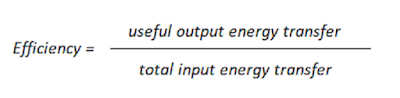# Work, power and efficiency

In this chapter you will learn:

1. Work, power and efficiency

2. What is wasted energy?

3. How to reduce unwanted transfer of energy?

## Work

When a force acts on an object and causes it to move through a distance, then work is being done on the object by the force.

When work is done, energy is transferred from one energy store to another.

work done = Energy transferred

work done = force × distance

The units used in the equation above are as follows:

• Work done (energy transferred) is measured in joules, J

• force is measured in newtons, N

• Distance is measured in metres, m

Worked example:

Question 1

A horizontal force of 30 N causes an object to move a horizontal distance of 20 m.

How much work is done on the object by the force?

work done = force × distance

work done = 30 × 20

work done = 600 J

## Power

Power is defined as the rate at which energy is transfered, or the rate at which work is done.

Power is measured in watts, W.

One watt is equal to one joule per second (J/s). This means that for every extra joule that is transferred per second, the power increases by one watt.

power = work done ÷ time

The units used in the equation above are as follows:

• power is measured in watts, W

• work done is measured in joules, J

• time is measured in seconds, s

Worked example:

Question 1

An electrical appliance transfers 24,000 J of energy in one minute. What is the power rating of the appliance?

power = work done ÷ time

power = 24,000 ÷ 60

power = 400 w

## Efficiency

Efficiency is a measurement of how much energy is transferred usefully.A more efficient device will waste very little of its input energy.

A very inefficient device will waste most of its input energy.

Efficency is commonly specified as percentage. Once you have calculated efficency using the equation above, multply it with 100 to get the percentage efficency.

Note: The maximum possible value of efficency is 100%. You cannot achieve an efficency greater than 100%.

For example, a motorbike that is 70% efficient, will turn 70% of its energy into movement and 30% into noise and heat.

Worked examples:

Question 1

The leaf blower is powered by an electric motor connected to a battery. The total power input to the leaf blower is 300 W. The useful power output of the leaf blower is 150 W.

Calculate the efficiency of the leaf blower?efficiency = useful output energy transfer ÷ total input energy transfer

efficency = 150 ÷ 300

efficency = 0.5 (or 50%)

Question 2

A 30W light bulb uses 400 J of electrical energy in a certain period of time. In that time, it produces 280 J of light energy. The rest of the energy is wasted.

Calculate the efficiency of this light bulb?efficiency = useful output energy transfer ÷ total input energy transfer

efficency = 280 ÷ 400

efficency = 0.7 (or 70%)

## Wasted energy

The three main ways in which devices waste energy are: Friction between their moving parts, electrical resistance and unwanted sound energy. The more efficient a process is, the less the amount of wasted energy.

For example, a light bulb produces light, but also produces heat energy, which is dissipated into the surroundings.

## Reducing unwanted transfer of energy

• Frictional forces can be reduce by lubricating the surfaces. Lubricant is a class of oils used to reduce the friction.

• Energy loss can be reduced using cavity wall insulation.

• Energy loss can be reduced by laying loft insulation.

• Energy loss can be reduced by using energy efficient devices, such as LED lamps.